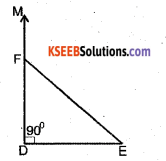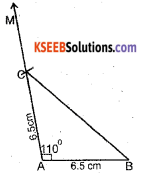# KSEEB Solutions for Class 7 Maths Chapter 10 Practical Geometry Ex 10.3

Students can Download Chapter 10 Practical Geometry Ex 10.3, Question and Answers, Notes Pdf, KSEEB Solutions for Class 7 Maths, Karnataka State Board Solutions help you to revise complete Syllabus and score more marks in your examinations.

## Karnataka State Syllabus Class 7 Maths Chapter 10 Practical Geometry Ex 10.3

Question 1.
Construct ∆ DEF such that DE = 5 cm, DF = 3 cm and ∠EDF = 90°.
Solution:Steps of Construction

1. Draw a line segment DE of length 5 cm. At D draw DM making 90° with DE.
2. With ‘D’ as centre, draw an arc of radius 3 cm, It cuts DM at the point F.
3. Join FE. The required triangle is obtained. That is ∆ DEF.Question 2.
Construct an Isosceles triangle in which the lengths of each of its equal sides is 6.5 cm and the angle between them is 110°.Solution:
Steps of Construction

1. Draw a line segment AB of length 6.5 cm. At A draw AM making 110° with AB using a protractor.
2. With ‘A’ as centre draw an arc of radius 6.5 cm and it cuts AM at C.
3. Join BC. The required triangle is ∆ ABC.Question 3.
Construct ∆ ABC with BC = 7.5 cm. AC = 5 cm and ∠C = 60°.
Solution:Steps of Construction

1. Draw a line segment BC of length 7.5 cm. At C draw CM making 60° with BC.
2. With ‘C’ as centre, draw an arc of radius 5cm, it cuts at CM at A.
3. Join AB. ∆ ABC is the required triangle.
error: Content is protected !!